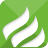3165538人观看发表
• 😁
• 😁
• 😂
• 🤣
• 😃
• 😄
• 😄
• 😅
• 😆
• 😉
• 😊
• 😋
• 😎
• 😍
• 😘
• 😗
• 😙
• 😚
• 🙂
• 🤗
• 🤔
• 😐
• 😑
• 😶
• 🙄
• 😏
• 😣
• 😥
• 😮
• 🤐
• 😯
• 😪
• 😫
• 😴
• 😌
• 😛
• 😜
• 😝
• 🤤
• 😒
• 😓
• 😔
• 😕
• 🙃
• 🤑
• 😲
• 😖
• 😞
• 😟
• 😤
• 😢
• 😭
• 😦
• 😧
• 😨
• 😩
• 😬
• 😰
• 😱
• 😳
• 😵
• 😡
• 😠
• 😷
• 🤒
• 🤕
• 🤢
• 🤧
• 😇
• 🤠
• 🤡
• 🤥
• 🤓
• 😈
• 👿
• 👹
• 👺

“亲情中华”主题活动自开展以来，已先后在全球80多个国家和地区的300座城市开展慰问演出，深受广大侨界群众和海外侨胞的欢迎和喜爱，成为中国侨联弘扬华夏文化、传播中国声音的一张亮丽名片。在中国共产党成立100周年之际，来自首都北京的艺术家们将为我们展现一桌精彩的艺术大餐。歌以咏志、乐以抒怀，一曲曲经典将穿越悠悠时空，凝聚起自贡侨界的拳拳赤子心、殷殷爱国情。请用手机观看
0.293777s